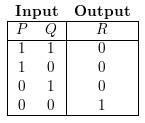Chapter 8.3, Problem 35ES### Discrete Mathematics With Applicat...

5th Edition
EPP + 1 other
ISBN: 9781337694193

#### Solutions

Chapter
Section### Discrete Mathematics With Applicat...

5th Edition
EPP + 1 other
ISBN: 9781337694193
Textbook Problem
1 views

# Find an additional representative circuit for the input/output table of Example 8.3.9.

To determine

An additional representative circuit for the input/output table given below.Explanation

Given information:

Calculation:

We then note that output is 1 if both inputs are 0.

Thus the input is 0 if P and Q are both 1 (PQ), if P is 1 and Q is 0 (PQ) or if P is 0 and Q is 1 (PQ).

Input=0(PQ)(Px

### Still sussing out bartleby?

Check out a sample textbook solution.

See a sample solution

#### The Solution to Your Study Problems

Bartleby provides explanations to thousands of textbook problems written by our experts, many with advanced degrees!

Get Started